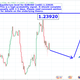# FIRST SELL equilibrium level for EURUSD!

Short
FX:EURUSD   Euro / U.S. Dollar
256 views
The Local Equilibrium level for a financial instrument X can be determined by a complex-analytic function E_x^t=f(A,B,C,D,E) WHERE
A:= measures the minimum probability that the instrument will be Stochastically-flexible within the next t-days.
B:= measures the class to which the financial instrument belongs. This is a number from 0 to 1.
C:= measures the probability that uncertain events will affect the fundamental state of the instrument-market.
D:= marginal propensity to fail.
E:= stochastic elasticity of the financial instrument.

This is a high-probability modern.
In the case of EURUSD WE obtain the value 1.22844 for the next equilibrium point!

Any up in EURUSD is a chance to sell!